# Log24

## Saturday, February 27, 2010

### Cubist Geometries

Filed under: General,Geometry — Tags: , — m759 @ 2:01 PM

"The cube has…13 axes of symmetry:
6 C2 (axes joining midpoints of opposite edges),
4 C3 (space diagonals), and
3C4 (axes joining opposite face centroids)."
–Wolfram MathWorld article on the cube

These 13 symmetry axes can be used to illustrate the interplay between Euclidean and Galois geometry in a cubic model of the 13-point Galois plane.

The geometer's 3×3×3 cube–
27 separate subcubes unconnected
by any Rubik-like mechanism–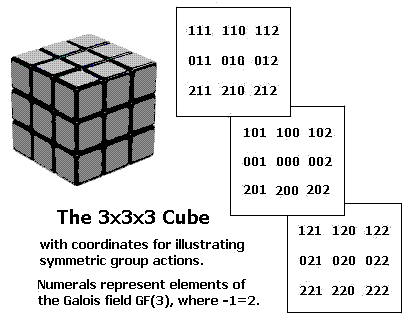The 13 symmetry axes of the (Euclidean) cube–
exactly one axis for each pair of opposite
subcubes in the (Galois) 3×3×3 cube–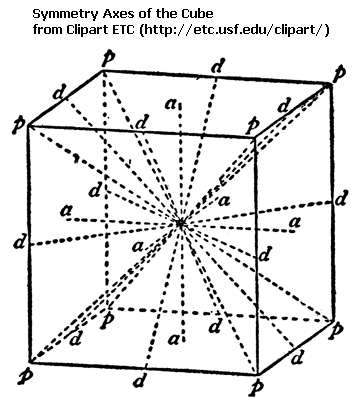A closely related structure–
the finite projective plane
with 13 points and 13 lines–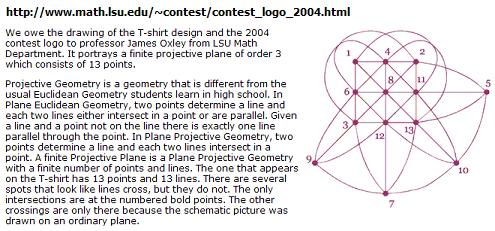A later version of the 13-point plane
by Ed Pegg Jr.–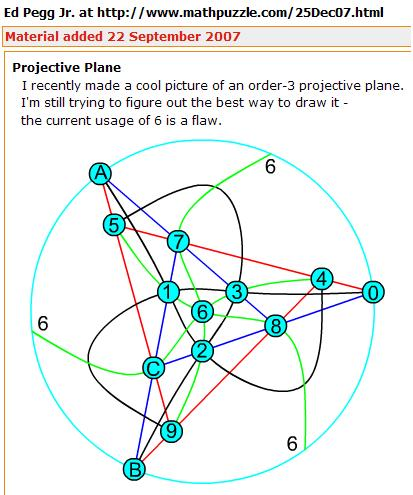A group action on the 3×3×3 cube
as illustrated by a Wolfram program
by Ed Pegg Jr. (undated, but closely
related to a March 26, 1985 note
by Steven H. Cullinane)–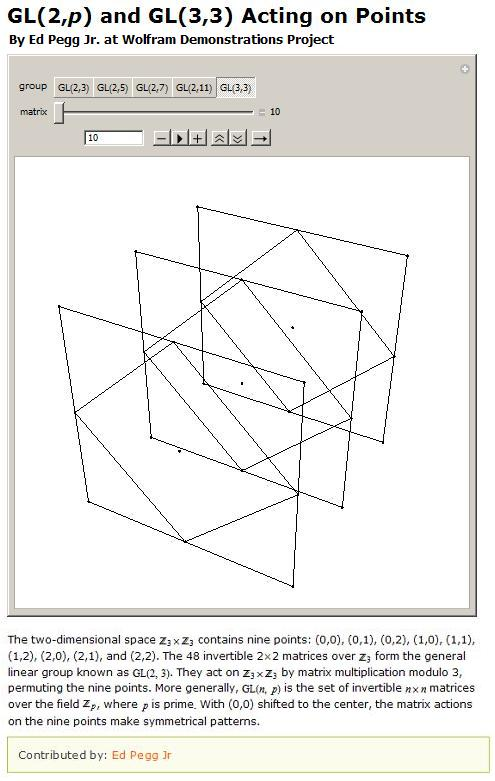The above images tell a story of sorts.
The moral of the story–

Galois projective geometries can be viewed
in the context of the larger affine geometries
from which they are derived.

The standard definition of points in a Galois projective plane is that they are lines through the (arbitrarily chosen) origin in a corresponding affine 3-space converted to a vector 3-space.

If we choose the origin as the center cube in coordinatizing the 3×3×3 cube (See Weyl's relativity problem ), then the cube's 13 axes of symmetry can, if the other 26 cubes have properly (Weyl's "objectively") chosen coordinates, illustrate nicely the 13 projective points derived from the 27 affine points in the cube model.

The 13 lines of the resulting Galois projective plane may be derived from Euclidean planes  through the cube's center point that are perpendicular to the cube's 13 Euclidean symmetry axes.

The above standard definition of points in a Galois projective plane may of course also be used in a simpler structure– the eightfold cube.

(The eightfold cube also allows a less standard way to picture projective points that is related to the symmetries of "diamond" patterns formed by group actions on graphic designs.)

See also Ed Pegg Jr. on finite geometry on May 30, 2006
at the Mathematical Association of America.